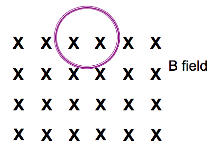# Problem: A circular loop of wire is positioned half in and half out of a square region of constant uniform magnetic field directed into the page (as shown in link below). State at least three things you can physically do to this wire or the magnetic field to induce a clockwise current in this loop. (Hint: What does electromagnetic induction depend on?)

###### FREE Expert Solution

$\overline{){\mathbf{V}}{\mathbf{=}}\frac{\mathbf{-}\mathbf{\Delta }\mathbf{\Phi }}{\mathbf{\Delta }\mathbf{t}}{\mathbf{=}}\frac{\mathbf{-}\mathbf{\Delta }\mathbf{\left(}\mathbf{B}\mathbf{A}\mathbf{c}\mathbf{o}\mathbf{s}\mathbf{\theta }\mathbf{\right)}}{\mathbf{\Delta }\mathbf{t}}}$

When one variable changes, the other two remain constant.

83% (171 ratings)###### Problem Details

A circular loop of wire is positioned half in and half out of a square region of constant uniform magnetic field directed into the page (as shown in link below). State at least three things you can physically do to this wire or the magnetic field to induce a clockwise current in this loop. (Hint: What does electromagnetic induction depend on?)